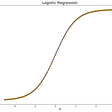# Linear Regression in Python using SciKit Learn

Originally published here: http://blog.contactsunny.com/data-science/linear-regression-in-python-using-scikit-learn

Today we’ll be looking at a simple Linear Regression example in Python, and as always, we’ll be using the SciKit Learn library. If you haven’t yet looked into my posts about data pre-processing, which is required before you can fit a model, checkout how you can encode your data to make sure it doesn’t contain any text, and then how you can handle missing data in your dataset. After that you have to make sure all your features are in the same range for the model so that one feature is not dominating the whole output; and for this, you need feature scaling. Finally, split your data into training and testing sets.

Once you’re done with all that, you’re ready to start your first and the most simple machine learning model, Linear Regression.

For this example, we’re going to use a different dataset than the one we’ve been using in our previous posts. This one is a bit bigger than that. The dataset has two columns — number of years of work experience, and the salary for that experience. Our goal is to build a model which will learn on this dataset and will be able to predict the salary for a given number of years of experience.

First, let’s import all the libraries and classes we need. We have a couple of new libraries/classes that we’ve not yet used, one for the linear regression model itself, and the other for plotting our results.

`import numpyimport matplotlib.pyplot as plotimport pandasfrom sklearn.model_selection import train_test_splitfrom sklearn.linear_model import LinearRegression`

We’re using a library called the ‘matplotlib,’ which helps us plot a variety of graphs and charts so that we can visualise our results easily.

After this, we import our dataset using the read_csv() method provided by pandas. We then separate the features and the dependent variable into variables x and y respectively. Because our data is all numbers and there’s no text in it, we don’t have to label encode or one hot encode our data. And because we’re using the LinearRegression class, we don’t even have to worry about feature scaling, as this is taken care of by the library itself.

So as soon as we separate the features and the dependent variable, we can split our data into training and test sets. The code for doing this is given below:

`# Import the datasetdataset = pandas.read_csv('salaryData.csv')x = dataset.iloc[:, :-1].valuesy = dataset.iloc[:, 1].values# Split the dataset into the training set and test set# We're splitting the data in 1/3, so out of 30 rows, 20 rows will go into the training set,# and 10 rows will go into the testing set.xTrain, xTest, yTrain, yTest = train_test_split(x, y, test_size = 1/3, random_state = 0)`

The next step for us is to simply create a linear regression object, fit it to our training set, and start predicting. But before that, if you want to understand the math behind linear regression and how it works, checkout this post.

Let’s now begin with creating our linear regression object. But before we do, let me tell you, thanks to libraries such as SciKit, we don’t really have to worry about the math behind all these algorithms. These libraries make lives easier by just exposing APIs to us so that we can call those APIs and get our results. We can tune the models, of course. But it is always better to have the knowledge of how a model works internally, so that if required, you can tune it better for your requirements.

With that out of the day, let’s create our object:

`linearRegressor = LinearRegression()`

And that’s pretty much how you create a linear regression model using SciKit. Next, we have to fit this model to our data, in other words, we have to make it “learn” using our training data. For that, its just one other line of code:

`linearRegressor.fit(xTrain, yTrain)`

Now, your model is trained with the training set you created. You can now start testing the model with the testing dataset you have. For that, you add one more line to your code:

`yPrediction = linearRegressor.predict(xTest)`

That’s pretty much it. Your linear regression model is ready! You can now celebrate.

The next step is to see how well your prediction is working. For this, we’ll use the MatPlotLib library. First, we’ll plot the actual values from our dataset against the predicted values for the training set. This will tell us how accurate our model is. After that, we’ll make another plot with the test set. In both cases, we’ll be using a scatter plot. We’ll plot the actual values (from the dataset) in red, and our model’s predictions in blue. This way, we’ll be able to easily differentiate the two. Let’s start with the code for plotting the training set:

`plot.scatter(xTrain, yTrain, color = 'red')plot.plot(xTrain, linearRegressor.predict(xTrain), color = 'blue')plot.title('Salary vs Experience (Training set)')plot.xlabel('Years of Experience')plot.ylabel('Salary')plot.show()`

It’s pretty easy, as you can see. And the plot looks like this:

As you can see, the red dots are near the blue line. But there’s definitely some variance. We can tune the model using a variety of techniques, but we’ll keep that for another day.

Now let’s look at the plot for the test set, and the code for that is here:

`plot.scatter(xTest, yTest, color = 'red')plot.plot(xTrain, linearRegressor.predict(xTrain), color = 'blue')plot.title('Salary vs Experience (Test set)')plot.xlabel('Years of Experience')plot.ylabel('Salary')plot.show()`

It’s the same code, we just change the variables for the red scatter plot. The graph looks like this:

The predictions are pretty close to the actual plot, which means the variance is pretty less. But we can definitely achieve more accuracy, as I already mentioned. We should also keep in mind that we only have one feature in our dataset, and having more features will definitely improve accuracy.

If you’re interested in looking at the dataset or the complete code, you can have a look at my Data Science Examples repository on GitHub.

Follow me on Twitter for more Data Science, Machine Learning, and general tech updates. Also, you can follow my personal blog as I post a lot of my tutorials, how-to posts, and machine learning goodness there before Medium.

If you like my posts here on Medium or on my personal blog, and would wish for me to continue doing this work, consider supporting me on Patreon.

--

--

--

## More from Sunny Srinidhi

Love podcasts or audiobooks? Learn on the go with our new app.

## MetaHeuristic For Hyper Parameter Tuning Using Python## EZlytix vs Tableau Comparison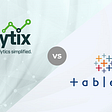## Value Stream Mapping in Agile Business Analysis## Data Storytelling — Basic Data Visualization in Excel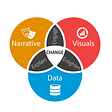## Essential Python Every Data Scientist Should Know In 2021## Custom Dashboards: Why Are They so Helpful?## Fraud Detection — Model Evaluation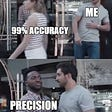## Machine Learning: Predicting used car prices## Simple Linear Regression From Scratch Using Python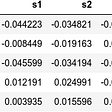## Polynomial Regression — Python Implementation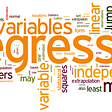## Logistic Regression ,the Sigmoid Function and its Application!